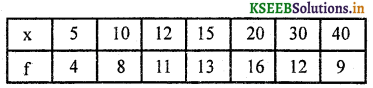# KSEEB Solutions for Class 8 Maths Chapter 13 Statistics Ex 13.3

Students can Download Class 8 Maths Chapter 13 Statistics Ex 13.3 Questions and Answers, Notes Pdf, KSEEB Solutions for Class 8 Maths helps you to revise the complete Karnataka State Board Syllabus and to clear all their doubts, score well in final exams.

## Karnataka State Syllabus Class 8 Maths Chapter 13 Statistics Ex 13.3

Question 1.
Runs scored by 10 batsman in a one day cricket match are given. Find the average run scored. 23, 54, 08, 94, 60, 18, 29, 44, 05, 86
Σ x = 23 + 54 + 08 + 94 + 60 + 18 + 29 + 44 + 05 + 86 = 421
N = 10
Average = Mean = $$\overline{X}=\frac{\Sigma x}{N}=\frac{421}{10}=42.1$$Question 2.
Find the mean weight form the following table:Mean =$\frac{\Sigma f_{X}}{N}=\frac{473}{15}=31.53$

Question 3.
Calculate the mean for the following frequency distribution.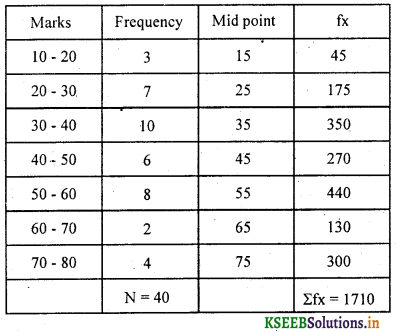Mean =$\frac{\Sigma f_{x}}{N}=\frac{1710}{40}=42.75$Answer:Question 4.
Calculate the mean for the following frequency distribution.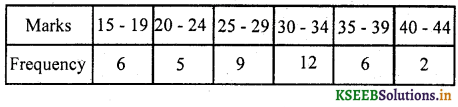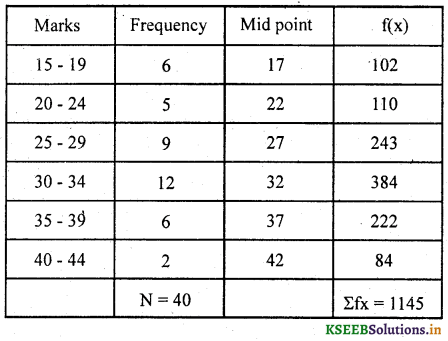Mean =$\overline{X}=\frac{\Sigma f_{X}}{N}=\frac{1145}{40}=28.625$

Question 5.
Find the median of the data 15,22, 9,20, 6,18,11,25,14.
6, 9, 11, 14,(15), 18, 20, 22,25 (Ascending order)
N = 9,
$$\frac{N+1}{2}+\frac{9+1}{2}=\frac{10}{2}=5^{\text { th }}$$
Median = 15.Question 6.
Find the median of the data 22, 28, 34, 49, 44, 57, 18, 10, 33, 41, 66, 59
10, 18, 22, 28, 33, 34, 41, 44, 49, 57, 59, 66 (Ascending order)
N = 10
∴ Median = $$\frac{34+41}{2}=\frac{75}{2}=37.5$$

Question 7.
Find the median for the following frequency distribution table.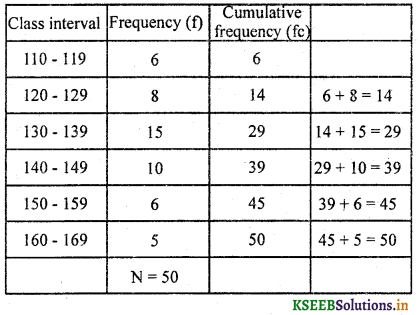N = 50$\frac{\mathrm{N}}{2}=\frac{50^{25}}{\not 2}=25$
∴ Median class is 130 – 139
LRL = 129.5
Fc = 14
Fm = 15
i = 10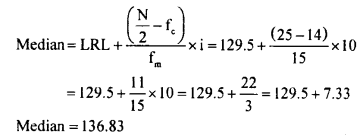Question 8.
Find the median for the following frequency distribution table.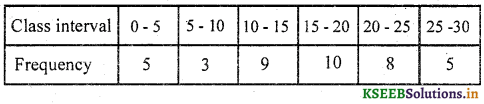N = 40$\frac{N}{2}=\frac{40}{2}=20$
∴ Median class is 15 – 20
LRL = 15
Fc = 17
Fm = 9
i = 5Question 9.
Find the mode for the following data.
(i) 4,3,1,5,3, 7, 9,6